# SAT Math Multiple Choice Question 70: Answer and Explanation

### Test Information

Question: 70

10.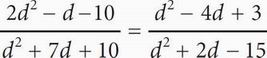In the equation above, what is the value of d ?

• A. -4
• B. 2
• C. 4
• D. 6

CWhenever the question includes variables and the answer choices are numbers, think Plugging In the Answers. In (A), d = -4, and the equation becomes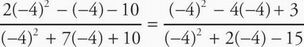. Solve both sides of the equation to get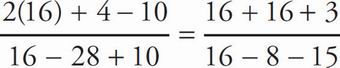, or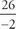=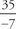. Reduce both fractions to get -13 = -5. This is not true, so eliminate (A). In (B), d = 2, and the equation becomes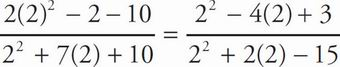. Solve both sides of the equation to get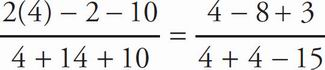, or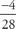=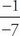. Reduce both fractions to get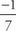=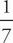. Eliminate (B). In (C), d = 4 and the equation becomes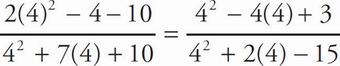. Solve both sides of the equation to get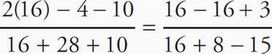, or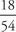=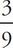. Reduce both fractions to get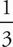=. The correct answer is (C).